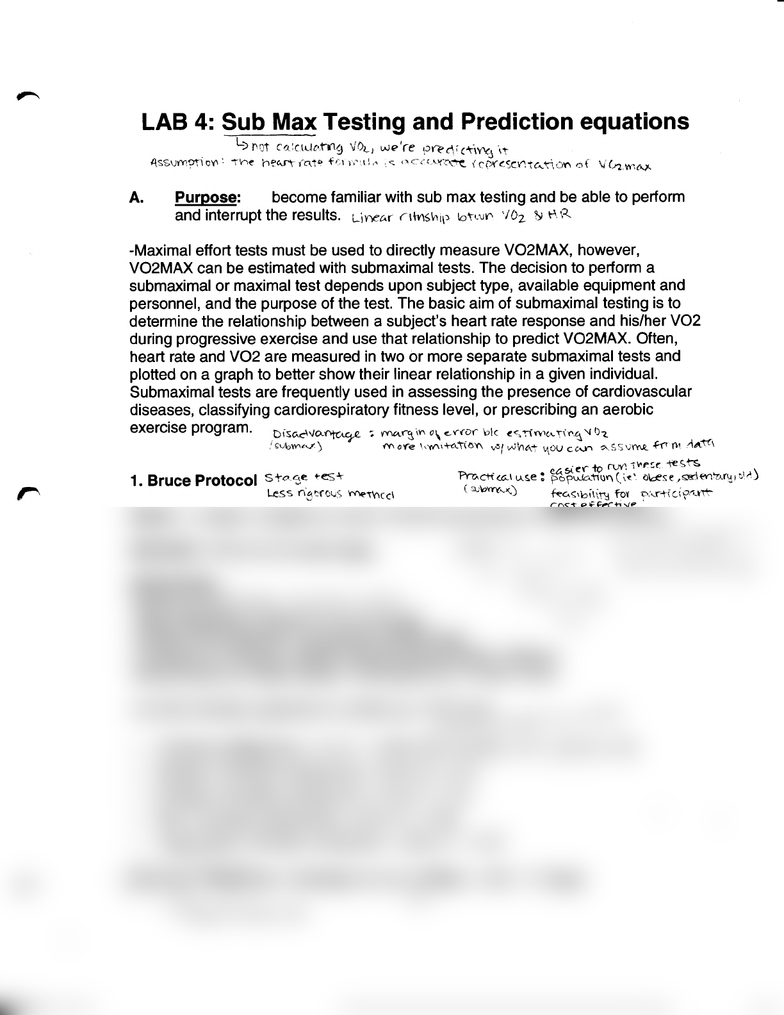Study Guides (400,000)
US (230,000)
Rutgers (3,000)
7:17 (70)
Final

# 01:377:371 Lecture Notes - Lecture 4: Cardiorespiratory Fitness, Joule, Tamil EelamExam

Department
Exercise Scienc
Course Code
01:377:371
Professor
Study Guide
Final

This preview shows half of the first page. to view the full 1 pages of the document.LAB
4:
Sub Max Testing and Prediction equations
.
L)rot
ca:ctdc-h1g
\fO~._.,
we<>'re
erec\'c'riY't'J
\'t
A.ssvm':)tloY'I:
...,...,...,~
h~"-f't{nt<>
+~~~
l•''l:,,
.
..c::
'"::-,·c•-1(::-\~
1c~i'.~O":"t~'t\OY'I
of
\i(.J?.Wlr'-1-
A.
Purpose: become familiar with sub max testing and be able to perform
and interrupt the results.
Lil"'\ec.r
nms\'il)
\o\l\JY'·
'ID
2
~
~-'
R
-Maximal effort tests must be used to directly measure V02MAX, however,
V02MAX
can be estimated with submaximal tests. The decision to perform a
submaximal or maximal test depends upon subject type, available equipment and
personnel, and the purpose of the test. The basic aim of submaximal testing is to
determine the relationship between a subject's heart rate response and his/her
V02
during progressive exercise and use that relationship to predict V02MAX. Often,
heart rate and
V02
are measured
in
two or more separate submaximal tests and
plotted
on
a graph to better show their linear relationship
in
a given individual.
Submaximal tests are frequently used
in
assessing the presence of cardiovascular
diseases, classifying cardiorespiratory fitness level, or prescribing an aerobic
exercise program.
_D,·sc..c::-~vo..~
-;
V'f\C.\.~\1'\
o\
~""?'
'l:>k
to:<,iiV'<'c"'\''~'-C:l
'~
')-z.
'
!;VIJIV'~~)
1Y\
o'('e
\•'ti\\+R't\OY\
....
~,
wint\"';" v1
0U
ctAn
?-
S S
\)~
~
f\-1
-'\<!'~:~t\
. e'f' to
ruv:
!VII'!:t:
-te
S.
'h:.
1. Bruce Protocol
st-olje.
+-es~
'Pr?Aeii~lt-~.s.e:
~~\.fJ<ATI~I"I(\~'
<>l.:£'se,oo€Yl~.n-!.,c',.l)
Less
n·8~·f<.:'-
..
6
'ffi(>\Yiccl
(
"JJ\>Yr{,><.)
f.t-c..<::,t:>ilin:!
fey o.\.r-t(c\'('"-Att
co~-t
effect\-..te
·
GOAL: To obtain complete as much of the Bruce protocol to
est1mate
V02 max.
RECORD: HR at end of each stage,
PROTOCOL:
-Warm up for 10 min
..->a;,vlc
t:J(:
s
VVlins
-Begin the Bruce protocol at the first stage,
-Monitor HR at the last 15 seconds of each stage
-Complete the protocol until the subject cannot safely continue
-Record time as a total number. Example 9 min 15 sec = 9.25
"'"e
c,f<:-
pt"'-ec\•<.~I'<'G
\ex\:+·:\
l _
_}
,.,r·.
t-\
~,
v-;Y~"""'
"';VVe
·.,
C-z.
-.;11\..IV~
wi\'
'ae
<'\."\.
1'\'!'...X.
Use the following equations to predict your
V02
max:
..._
c.eo)
.
(vJif1~.1"1e'-~e("
-res.'
.e'<\
'\d'l'
.....
V02max
(ml/kg/min) =
14.76-
(1.379
x~)
+ (0.451 x T2
)-
(0.012 x T3)
Women:
V02max
(ml/kg/min) = 2.94 x T + 3.74
Women:
V02max
(ml/kg/min) = 4.38 x T - 3.9
Men:
V02max
(ml/kg/min) = 2.94 x T + 7.65
Young Men:
V02max
(ml/kg/min) = 3.62 x T +
3.91
~ate~HRM~rom
following formula: HRMax =
208-
0.7(age)
L
34
>
I(Af'q
et-
\-\P.
ft.f
teSt